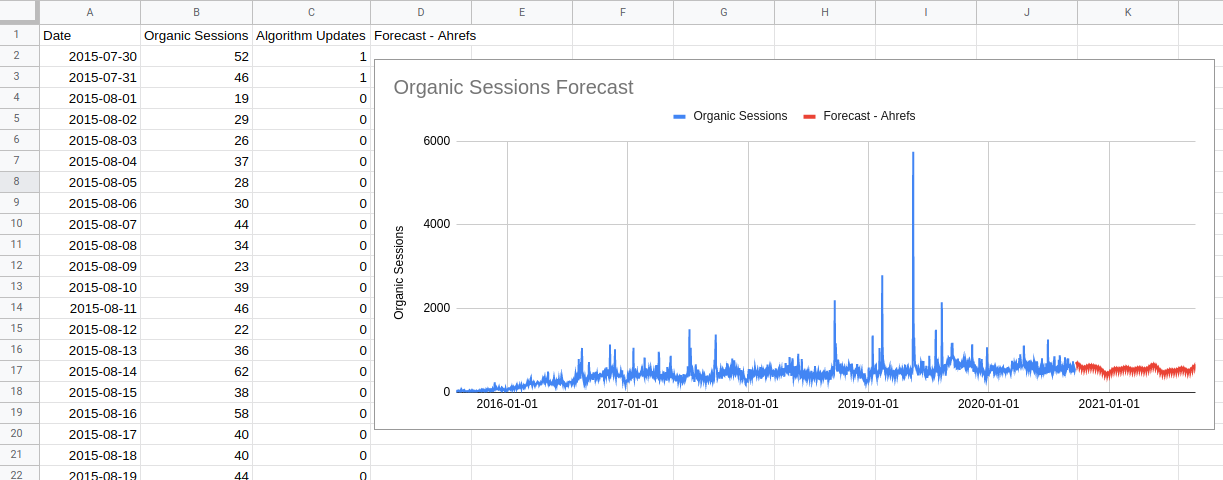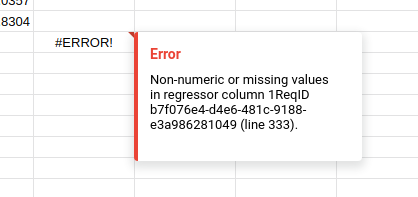# The Anvil## Extending Prophet for fun and profit - Part 2

October 27, 2020

This post is part of a series on extending the Prophet forecasting model so that it can do other things. You should read part one on the default Prophet forecasting model first.

## What Prophet Can’t Do

For me, one of the most useful features of Prophet is how easily you can add regression features to the model. This enables you to provide information to the machine learning model about things like the dates when email campaigns were sent or when your PPC budget increased; and you can use your marketing calendar to add information about when these things will happen in the future too.

Being able to do this in a spreadsheet is one of the main things that makes Forecast Forge great.

October 19, 2020

## Understanding the original implementation

Prophet is a general purpose forecast algorithm developed by Facebook. It is the main algorithm used behind the scenes for Forecast Forge (I’ll go into why I picked it some other time).

At the core of the Prophet code is a model written in a programming language called Stan. I am lucky enough to have some familiarity with Stan from other projects.

This model is where the interesting stuff happens; the rest of the Prophet code (R or python) is just around scaling and preparing data for input into this model and extracting the results at the end.

I’m going to assume for this that you are familiar with the process of using Prophet in R or Python. If you aren’t then read the getting started guide and fit a few forecasts. After that this all should all make a bit more sense.

### We need to talk about Stan

Suppose you have some process that you are interested in and that this process is complicated enough that you want to model it as something random or stochastic. Calculating how long a car traveling at 70 miles per hour takes to go between two towns doesn’t fit into this category (it is too easy to model without) but something like whether or not a user converts on a website is; it is just too much to take into account all the variables like their mental state, what they had for lunch, whether their neighbor is slowing down your site by watching Netflix at the same time as they surf and all the rest.

Stan let’s you describe how this random process works (called a “generative model”) and then does some clever stuff in the background to figure out the parameters of the model. For example, a simple model for website conversion might be “the user converts at random with probability `p` and does not convert with probability `1-p`”. Then Stan does Bayesian inference with this model and your data to tell you what the likely values of `p` are.

You might think this is a lot of work for something like estimating a conversion rate and you’d be right. Stan is more useful when the model gets more complicated and I will show you an example of this in this post.

### The Prophet model

In this post I will go through the existing Prophet Stan model. Then, in future posts I will discuss how it can be changed and modified for different things.

You can view the current model on github.

Stan is kind of an old fashioned language in that variables need to be declared in advance and there is very little flexibility in the order of code. Rather than start at the top and work down we will start at the end - at the absolute key part - and then work out.

#### The model

The most important part of the model is on line 136:

``````y ~ normal(
trend
.* (1 + X * (beta .* s_m))
+ X * (beta .* s_a),
sigma_obs
);``````

This describes how the `y` values (i.e. the thing we are trying to forecast) vary depending on the things they depend on.

If I rewrite it like this it should be easier to see what is going on:

``````y ~ normal(
trend
.* multiplicative_regressors
sigma_obs
);``````

So the `y` values are normally distributed around a `trend`, multiplied by some `multiplicative_regressors` and added to some `additive_regressors`. The variance is `sigma_obs`; a small value of `sigma_obs` will mean the model fits the `y` values used in training very closely so the predictive interval for the forecasted values will be narrower. `sigma_obs` is estimated from the data during model fitting.

Let’s unpack the regressors a bit more. Both of them have the form `X * (beta .* s_?)` where `s_?` is either `s_a` or `s_m`.

The `s_?` variables are provided by the user when they specify for each regressor whether it is multiplicative or additive. Each `s_?` is a vector with the same length as the number of regressors. For regressor number `i`, `s_a[i]` is `1` if it is an additive regressor and `s_m[i]` is `1` if it is a multiplicative regressor.

The `beta` values are regression coefficients. In Stan `.*` means elementwise multiplication so element `i` in the vector `beta .* s_a` is `beta[i] * s_a[i]`.

Because of how we defined `s_a` earlier this means it is zero unless it is an additive regressor. So `X * (beta .* s_a)` means only the additive regressors are included in the additive component. The same goes for the multiplicative regressors as `s_m`.

`X` is a matrix containing the values of the regressor variables; again, the user provides this before fitting starts.

If this looks very similar to the maximum likelihood method for doing a regression that is because it is! One of the things that amazes me about Prophet is that it can work so well across so many different things with such a simple model.

I guess “just use regression!” is a meme for a reason.## Video: Picking a data transform

October 16, 2020

Data transforms are super important for getting the most out of your forecasting. With Forecast Forge you need to use them if your data must be within a specific range (e.g. sessions must always be greater than zero, conversion rate must be between 0% and 100%) and they can also improve the accuracy of your forecasts too!

This video shows you how to test different data transformations and pick the one that performs the best.

All the examples in this video use the sidebar to configure the forecast rather than the `FORGE_FORECAST` custom function. This is because the using the sidebar enables us to automatically backtest the forecast method against historical data. You can do this with `FORGE_FORECAST` too but it is more effort.

## A Forecasting Template with SEO Algorithm Updates

September 21, 2020

I recently read a very interesting post from Patrick Stox on the Ahrefs blog. Since it concerns itself with SEO forecasting I wanted to write something covering two main points:

1. How to do this in Forecast Forge
2. Different (perhaps better?) methods for dealing with algorithm updates

Patrick uses a forecasting algorithm which is part of an open source project called Prophet. Prophet was created by Facebook and it is the main algorithm used by Forecast Forge (at the time of writing). It is possible to produce a very similar forecast to what Patrick/Ahrefs have done using Forecast Forge.

You can see an example template of this in Google Sheets## Post-Bank Holiday Update

September 1, 2020

This last week has been all about usability.

I have tried to improve the error messages so that when the inputs are bad or something else goes wrong you have a better idea of what it is and how to fix it.Believe it or not, this is way better than it was before

There is still a lot of work to be done here but these improved messages will hopefully help people use the tool; an important part of learning is making mistakes and experimenting but this doesn’t work if you can’t get good feedback - if you are just guessing and hoping for the best it will take a lot longer to learn.